# Electrical and Electronics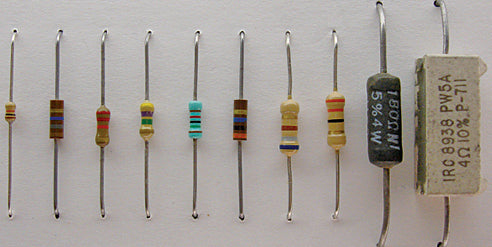### What is a Resistor?

Dive into the world of resistors! Discover their types, how to choose the right one, and their diverse applications in electronics.

### What is a Resistor?

Dive into the world of resistors! Discover their types, how to choose the right one, and their diverse applications in electronics.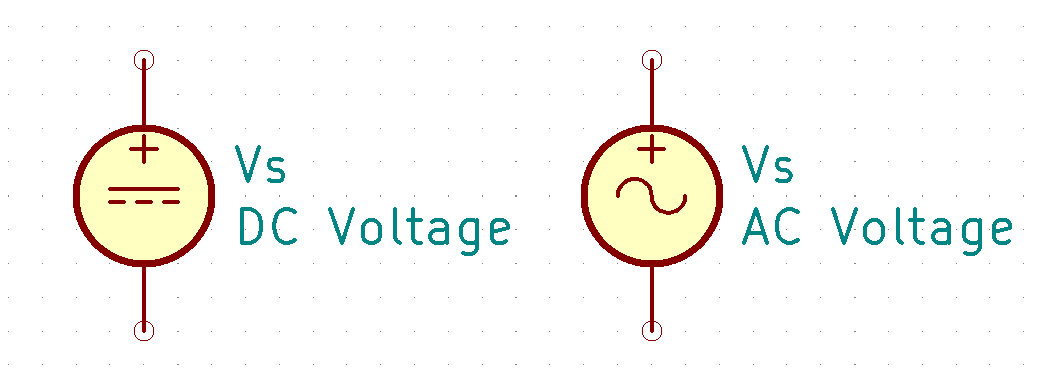### Voltage Demystified: A Deep Dive into Electrica...

Introduction Welcome to All About Electrical and Electronics, where we dive into the captivating realm of STEM and DIY projects! In this article, we embark on a journey to unravel...

### Voltage Demystified: A Deep Dive into Electrica...

Introduction Welcome to All About Electrical and Electronics, where we dive into the captivating realm of STEM and DIY projects! In this article, we embark on a journey to unravel...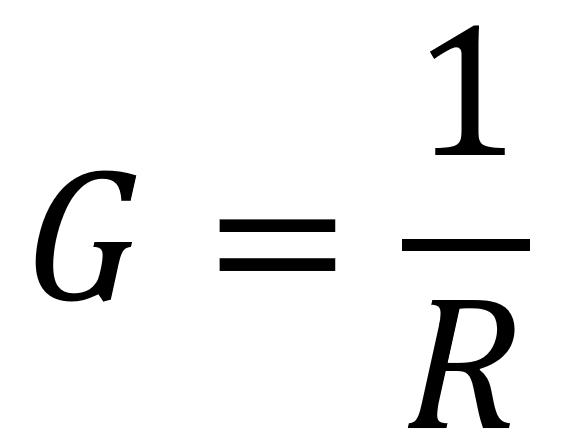### Conductance Formula

Introduction Conductance    The reciprocal of resistance is conductance, symbolized by . It is a measurement of the ease, with which current is established. The formula is The unit of conductance...

### Conductance Formula

Introduction Conductance    The reciprocal of resistance is conductance, symbolized by . It is a measurement of the ease, with which current is established. The formula is The unit of conductance...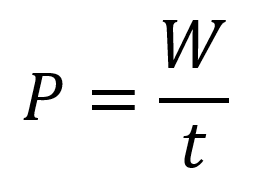### Energy & Power Formula

0.0 Introduction Energy is the ability to do work, and power is the rate at which energy is used. Power ( ) is a certain amount of energy ( )...

### Energy & Power Formula

0.0 Introduction Energy is the ability to do work, and power is the rate at which energy is used. Power ( ) is a certain amount of energy ( )...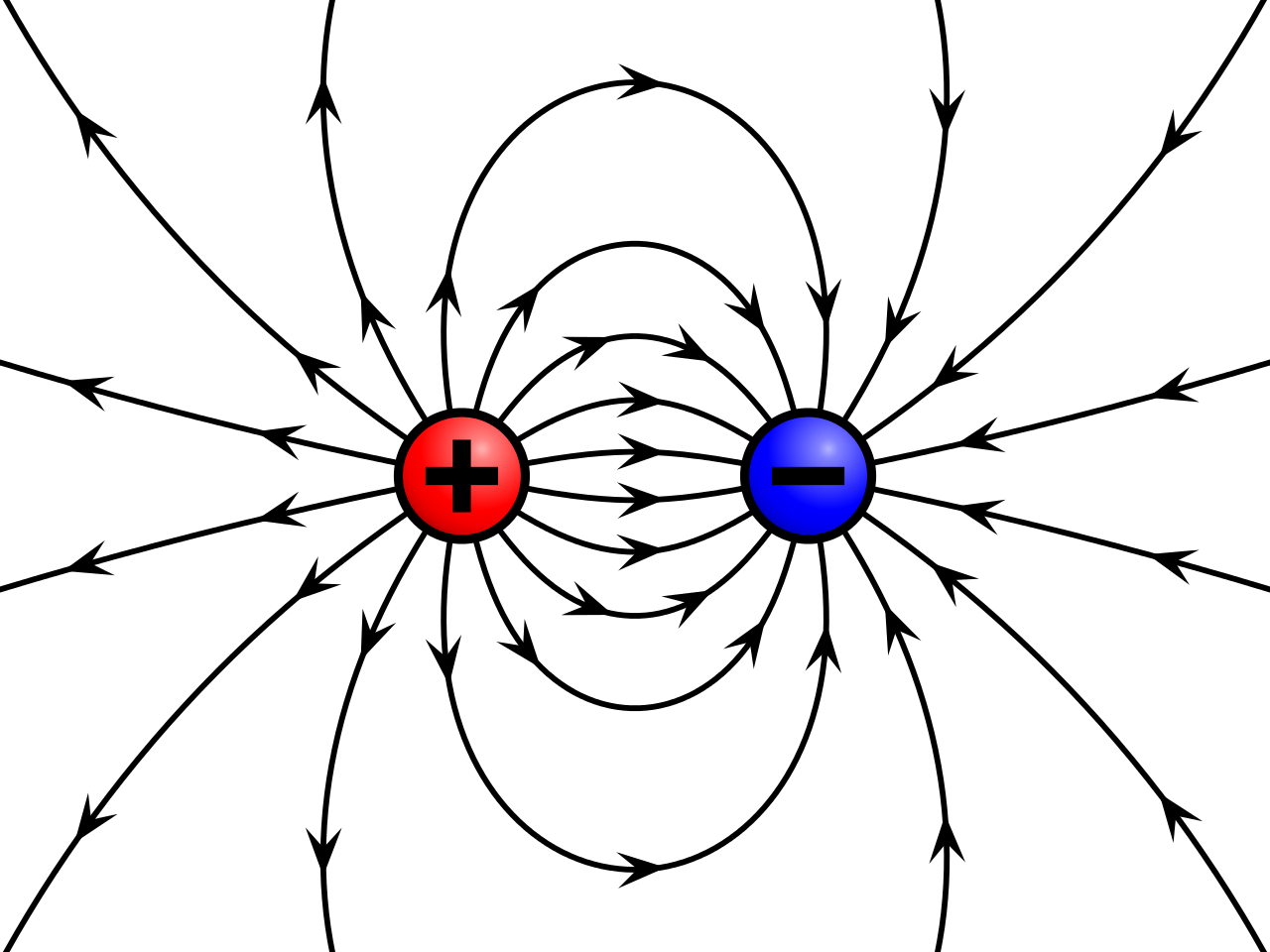### What Is An Electrical Charge?

The charge of an electron and that of a proton are equal in magnitude. Electrical charge is an electrical property of matter that exists because of an excess or deficiency of electrons....

### What Is An Electrical Charge?

The charge of an electron and that of a proton are equal in magnitude. Electrical charge is an electrical property of matter that exists because of an excess or deficiency of electrons....